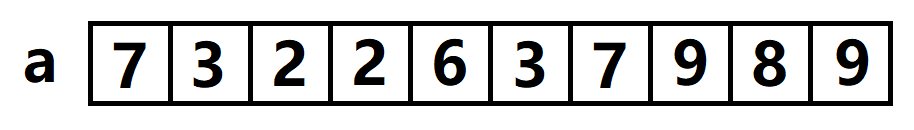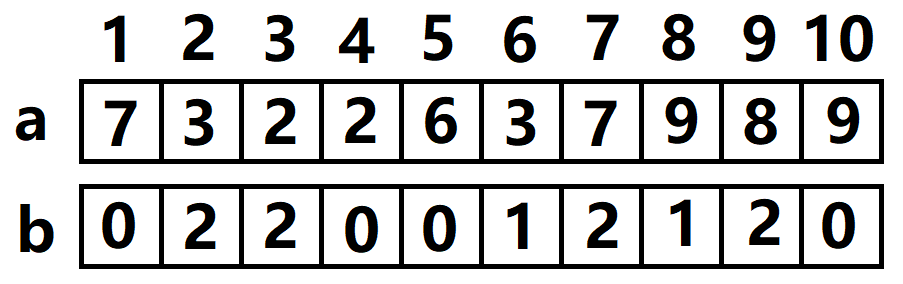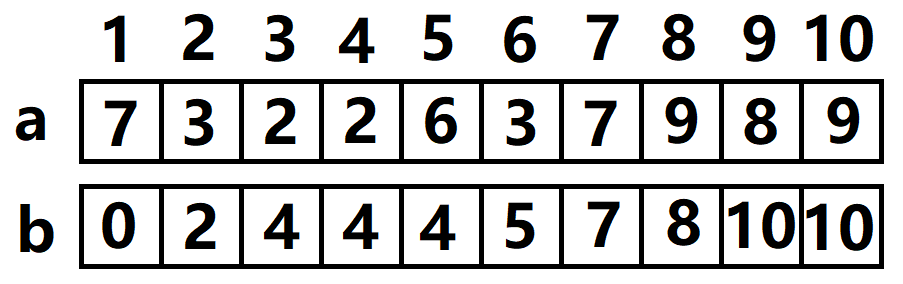# Ⅱ、分析问题

1、处理出数据中每个数出现的频率，放入数组$b$
2、处理处数据频率表的前缀形式，此时第$i$个位置的数据表示$a$数组的全部数据中，小于等于$a_i$的数量（包括a_i本身）
3、依照前缀表处理出排好序的数组#include<bits/stdc++.h>
#define F(i,j,n) for(register int i=j;i<=n;i++)
#define INF 0x3f3f3f3f
#define ll long long
#define mem(i,j) memset(i,j,sizeof(i))
using namespace std;
int n,m,a,b,c;
int datta=0;char chchc=getchar();bool okoko=0;
while(chchc<'0'||chchc>'9'){if(chchc=='-')okoko=1;chchc=getchar();}
while(chchc>='0'&&chchc<='9'){datta=datta*10+chchc-'0';chchc=getchar();}
return okoko?-datta:datta;
}
int main(){
}